# Java Programming Tutorial

## OOP Exercises

### Exercises on Classes

#### An Introduction to Classes and Instances by Example - The Circle Class

This first exercise shall lead you through all the basic concepts in OOP.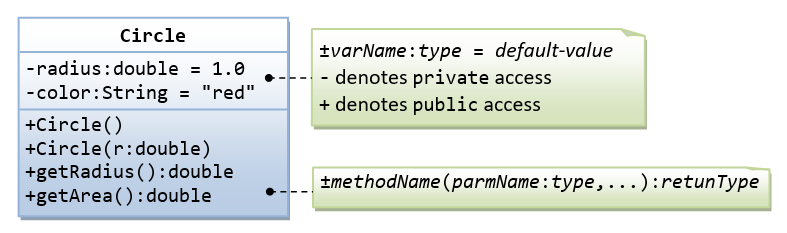A class called `circle` is designed as shown in the following class diagram. It contains:

• Two `private` instance variables: `radius` (of the type `double`) and `color` (of the type `String`), with default value of `1.0` and "`red`", respectively.
• Two overloaded constructors - a default constructor with no argument, and a constructor which takes a double argument for radius.
• Two `public` methods: `getRadius()` and `getArea()`, which return the radius and area of this instance, respectively.

The source codes for `Circle.java` is as follows:

```/**
* The Circle class models a circle with a radius and color.
*/
public class Circle {  // Save as "Circle.java"
// private instance variable, not accessible from outside this class
private String color;

/** Constructs a Circle instance with default value for radius and color */
public Circle() {  // 1st (default) constructor
color = "red";
}

/** Constructs a Circle instance with the given radius and default color */
public Circle(double r) {  // 2nd constructor
color = "red";
}

}

/** Returns the area of this Circle instance */
public double getArea() {
}
}```

Compile "`Circle.java`". Can you run the `Circle` class? Why?

This `Circle` class does not have a `main()` method. Hence, it cannot be run directly. This `Circle` class is a “building block” and is meant to be used in another program.

Let us write a test program called `TestCircle` (in another source file called `TestCircle.java`) which uses the `Circle` class, as follows:

```/**
*  A Test Driver for the Circle class
*/
public class TestCircle {  // Save as "TestCircle.java"
public static void main(String[] args) {
// Declare an instance of Circle class called c1.
// Construct the instance c1 by invoking the "default" constructor
// which sets its radius and color to their default value.
Circle c1 = new Circle();
// Invoke public methods on instance c1, via dot operator.
System.out.println("The circle has radius of "
+ c1.getRadius() + " and area of " + c1.getArea());
//The circle has radius of 1.0 and area of 3.141592653589793

// Declare an instance of class circle called c2.
// Construct the instance c2 by invoking the second constructor
// with the given radius and default color.
Circle c2 = new Circle(2.0);
// Invoke public methods on instance c2, via dot operator.
System.out.println("The circle has radius of "
+ c2.getRadius() + " and area of " + c2.getArea());
//The circle has radius of 2.0 and area of 12.566370614359172
}
}```

Now, run the `TestCircle` and study the results.

##### More Basic OOP Concepts
1. Constructor: Modify the class `Circle` to include a third constructor for constructing a `Circle` instance with two arguments - a `double` for `radius` and a `String` for `color`.
```// 3rd constructor to construct a new instance of Circle with the given radius and color
public Circle (double r, String c) { ...... }```
Modify the test program `TestCircle` to construct an instance of `Circle` using this constructor.
2. Getter: Add a getter for variable `color` for retrieving the `color` of this instance.
```// Getter for instance variable color
public String getColor() { ...... }```
Modify the test program to test this method.
3. public vs. private: In `TestCircle`, can you access the instance variable `radius` directly (e.g., `System.out.println(c1.radius)`); or assign a new value to `radius` (e.g., `c1.radius=5.0`)? Try it out and explain the error messages.
4. Setter: Is there a need to change the values of `radius` and `color` of a `Circle` instance after it is constructed? If so, add two `public` methods called setters for changing the `radius` and `color` of a `Circle` instance as follows:
```// Setter for instance variable radius
}

// Setter for instance variable color
public void setColor(String newColor) { ...... }```
Modify the `TestCircle` to test these methods, e.g.,
```Circle c4 = new Circle();   // construct an instance of Circle
c4.setColor("green");       // Change color
System.out.println("color is: " + c4.getColor());   // Print color via getter

// You cannot do the following because setRadius() returns void, which cannot be printed
5. Keyword "this": Instead of using variable names such as `r` (for `radius`) and `c` (for `color`) in the methods' arguments, it is better to use variable names `radius` (for `radius`) and `color` (for `color`) and use the special keyword "`this`" to resolve the conflict between instance variables and methods' arguments. For example,
```// Instance variable

/** Constructs a Circle instance with the given radius and default color */
// "radius" refers to the method's parameter
color = "red";
}

/** Sets the radius to the given value */
// "radius" refers to the method's argument
}```
Modify ALL the constructors and setters in the `Circle` class to use the keyword "`this`".
6. Method toString(): Every well-designed Java class should contain a `public` method called `toString()` that returns a description of the instance (in the return type of `String`). The `toString()` method can be called explicitly (via `instanceName.toString()`) just like any other method; or implicitly through `println()`. If an instance is passed to the `println(anInstance)` method, the `toString()` method of that instance will be invoked implicitly. For example, include the following `toString()` methods to the `Circle` class:
```/** Return a self-descriptive string of this instance in the form of Circle[radius=?,color=?] */
public String toString() {
}```
Try calling `toString()` method explicitly, just like any other method:
```Circle c5 = new Circle(5.5);
System.out.println(c5.toString());   // explicit call```
`toString()` is called implicitly when an instance is passed to `println()` method, for example,
```Circle c6 = new Circle(6.6);
System.out.println(c6.toString());  // explicit call
System.out.println(c6);             // println() calls toString() implicitly, same as above
System.out.println("Operator '+' invokes toString() too: " + c6);  // '+' invokes toString() too```

The final class diagram for the `Circle` class is as follows: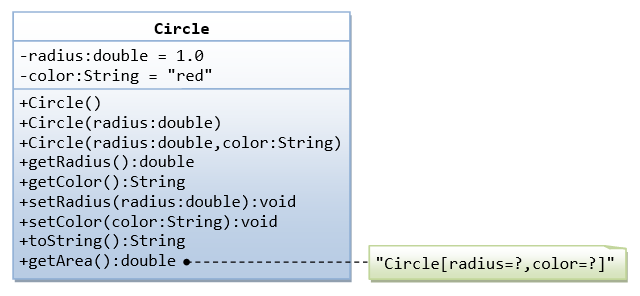#### Ex: Yet Another Circle Class

A class called `Circle`, which models a circle with a radius, is designed as shown in the following class diagram. Write the `Circle` class.Below is a Test Driver to test your `Circle` class.

```public class TestMain {
public static void main(String[] args) {
// Test Constructors and toString()
Circle c1 = new Circle(1.1);
System.out.println(c1);   // toString()
Circle c2 = new Circle(); // default constructor
System.out.println(c2);

// Test setter and getter
System.out.println(c1);      // toString()

// Test getArea() and getCircumference()
System.out.printf("area is: %.2f%n", c1.getArea());
System.out.printf("circumference is: %.2f%n", c1.getCircumference());
}
}```

The expected output is:

```Circle[radius=1.1]
area is: 15.21
circumference is: 13.82```

#### Ex: The Rectangle Class

A class called `Rectangle`, which models a rectangle with a length and a width (in `float`), is designed as shown in the following class diagram. Write the `Rectangle` class.Below is a test driver to test the `Rectangle` class:

```public class TestMain {
public static void main(String[] args) {
// Test constructors and toString()
// You need to append a 'f' or 'F' to a float literal
Rectangle r1 = new Rectangle(1.2f, 3.4f);
System.out.println(r1);  // toString()
Rectangle r2 = new Rectangle();  // default constructor
System.out.println(r2);

// Test setters and getters
r1.setLength(5.6f);
r1.setWidth(7.8f);
System.out.println(r1);  // toString()
System.out.println("length is: " + r1.getLength());
System.out.println("width is: " + r1.getWidth());

// Test getArea() and getPerimeter()
System.out.printf("area is: %.2f%n", r1.getArea());
System.out.printf("perimeter is: %.2f%n", r1.getPerimeter());
}
}```

The expected output is:

```Rectangle[length=1.2,width=3.4]
Rectangle[length=1.0,width=1.0]
Rectangle[length=5.6,width=7.8]
length is: 5.6
width is: 7.8
area is: 43.68
perimeter is: 26.80```

#### Ex: The Employee Class

A class called `Employee`, which models an employee with an ID, name and salary, is designed as shown in the following class diagram. The method `raiseSalary(percent)` increases the salary by the given percentage. Write the `Employee` class.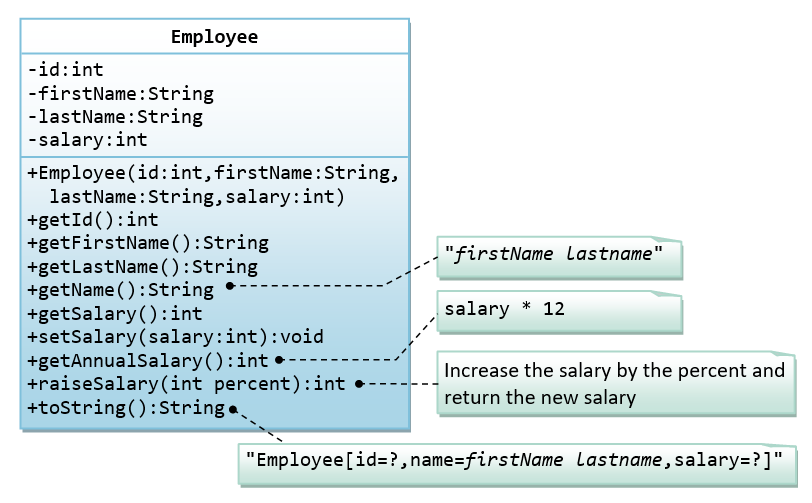Below is a test driver to test the `Employee` class:

```public class TestMain {
public static void main(String[] args) {
// Test constructor and toString()
Employee e1 = new Employee(8, "Peter", "Tan", 2500);
System.out.println(e1);  // toString();

// Test Setters and Getters
e1.setSalary(999);
System.out.println(e1);  // toString();
System.out.println("id is: " + e1.getId());
System.out.println("firstname is: " + e1.getFirstName());
System.out.println("lastname is: " + e1.getLastName());
System.out.println("salary is: " + e1.getSalary());

System.out.println("name is: " + e1.getName());
System.out.println("annual salary is: " + e1.getAnnualSalary()); // Test method

// Test raiseSalary()
System.out.println(e1.raiseSalary(10));
System.out.println(e1);
}
}```

The expected out is:

```Employee[id=8,name=Peter Tan,salary=2500]
Employee[id=8,name=Peter Tan,salary=999]
id is: 8
firstname is: Peter
lastname is: Tan
salary is: 999
name is: Peter Tan
annual salary is: 11988
1098
Employee[id=8,name=Peter Tan,salary=1098]```

#### Ex: The InvoiceItem Class

A class called `InvoiceItem`, which models an item of an invoice, with ID, description, quantity and unit price, is designed as shown in the following class diagram. Write the `InvoiceItem` class.Below is a test driver to test the `InvoiceItem` class:

```public class TestMain {
public static void main(String[] args) {
// Test constructor and toString()
InvoiceItem inv1 = new InvoiceItem("A101", "Pen Red", 888, 0.08);
System.out.println(inv1);  // toString();

// Test Setters and Getters
inv1.setQty(999);
inv1.setUnitPrice(0.99);
System.out.println(inv1);  // toString();
System.out.println("id is: " + inv1.getId());
System.out.println("desc is: " + inv1.getDesc());
System.out.println("qty is: " + inv1.getQty());
System.out.println("unitPrice is: " + inv1.getUnitPrice());

// Test getTotal()
System.out.println("The total is: " + inv1.getTotal());
}
}```

The expected output is:

```InvoiceItem[id=A101,desc=Pen Red,qty=888,unitPrice=0.08]
InvoiceItem[id=A101,desc=Pen Red,qty=999,unitPrice=0.99]
id is: A101
desc is: Pen Red
qty is: 999
unitPrice is: 0.99
The total is: 989.01
```

#### Ex: The Account Class

A class called `Account`, which models a bank account of a customer, is designed as shown in the following class diagram. The methods `credit(amount)` and `debit(amount)` add or subtract the given `amount` to the `balance`. The method `transferTo(anotherAccount, amount)` transfers the given `amount` from this `Account` to the given `anotherAccount`. Write the `Account` class.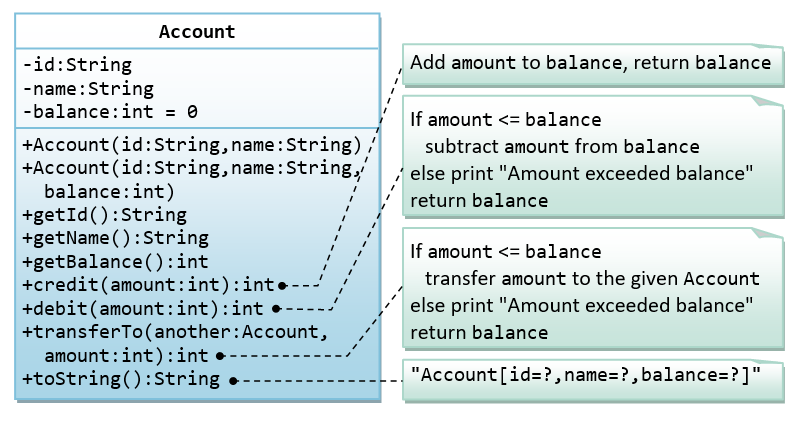Below is a test driver to test the `Account` class:

```public class TestMain {
public static void main(String[] args) {
// Test constructor and toString()
Account a1 = new Account("A101", "Tan Ah Teck", 88);
System.out.println(a1);  // toString();
Account a2 = new Account("A102", "Kumar"); // default balance
System.out.println(a2);

// Test Getters
System.out.println("ID: " + a1.getID());
System.out.println("Name: " + a1.getName());
System.out.println("Balance: " + a1.getBalance());

// Test credit() and debit()
a1.credit(100);
System.out.println(a1);
a1.debit(50);
System.out.println(a1);
a1.debit(500);  // debit() error
System.out.println(a1);

// Test transfer()
a1.transferTo(a2, 100);  // toString()
System.out.println(a1);
System.out.println(a2);
}
}```

The expected output is:

```Account[id=A101,name=Tan Ah Teck,balance=88]
Account[id=A102,name=Kumar,balance=0]
ID: A101
Name: Tan Ah Teck
Balance: 88
Account[id=A101,name=Tan Ah Teck,balance=188]
Account[id=A101,name=Tan Ah Teck,balance=138]
Amount exceeded balance
Account[id=A101,name=Tan Ah Teck,balance=138]
Account[id=A101,name=Tan Ah Teck,balance=38]
Account[id=A102,name=Kumar,balance=100]```

#### Ex: The Date Class

A class called `Date`, which models a calendar date, is designed as shown in the following class diagram. Write the `Date` class.Below is a test driver to test the `Date` class:

```public class TestMain {
public static void main(String[] args) {
// Test constructor and toString()
Date d1 = new Date(1, 2, 2014);
System.out.println(d1);  // toString()

// Test Setters and Getters
d1.setMonth(12);
d1.setDay(9);
d1.setYear(2099);
System.out.println(d1);  // toString()
System.out.println("Month: " + d1.getMonth());
System.out.println("Day: " + d1.getDay());
System.out.println("Year: " + d1.getYear());

// Test setDate()
d1.setDate(3, 4, 2016);
System.out.println(d1);  // toString()
}
}```

The expected output is:

```01/02/2014
09/12/2099
Month: 12
Day: 9
Year: 2099
03/04/2016```

#### Ex: The Time Class

A class called `Time`, which models a time instance, is designed as shown in the following class diagram. The methods `nextSecond()` and `previousSecond()` shall advance or rewind this instance by one second, and return this instance, so as to support chaining operation such as `t1.nextSecond().nextSecond()`. Write the `Time` class.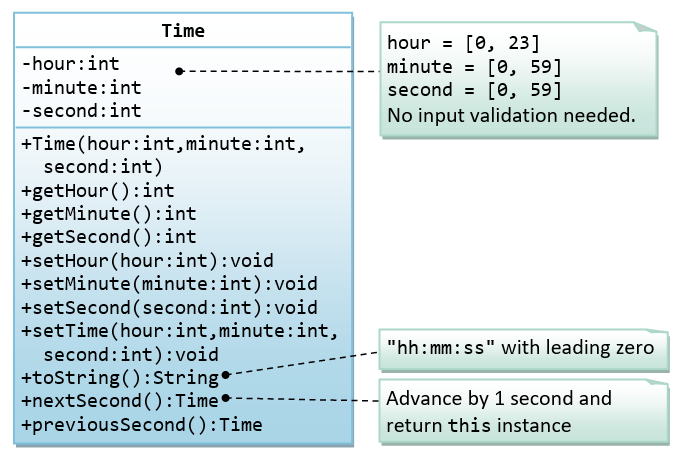Below is a test driver for testing the `Time` class:

```public class TestMain {
public static void main(String[] args) {
// Test constructors and toString()
Time t1 = new Time(1, 2, 3);
System.out.println(t1);  // toString()

// Test Setters and Getters
t1.setHour(4);
t1.setMinute(5);
t1.setSecond(6);
System.out.println(t1);  // toString()
System.out.println("Hour: " + t1.getHour());
System.out.println("Minute: " + t1.getMinute());
System.out.println("Second: " + t1.getSecond());

// Test setTime()
t1.setTime(23, 59, 58);
System.out.println(t1);  // toString()

// Test nextSecond();
System.out.println(t1.nextSecond());
System.out.println(t1.nextSecond().nextSecond());

// Test previousSecond()
System.out.println(t1.previousSecond());
System.out.println(t1.previousSecond().previousSecond());
}
}```

The expected output is:

```01:02:03
04:05:06
Hour: 4
Minute: 5
Second: 6
23:59:58
23:59:59
00:00:01
00:00:00
23:59:58```

#### Ex: The Ball Class

A class called `Ball`, which models a bouncing ball, is designed as shown in the following class diagram. It contains its radius, x and y position. Each move-step advances the x and y by delta-x and delta-y, respectively. delta-x and delta-y could be positive or negative. The `reflectHorizontal()` and `reflectVertical()` methods could be used to bounce the ball off the walls. Write the `Ball` class. Study the test driver on how the ball bounces.Below is a test driver:

```public class TestMain {
public static void main(String[] args) {
// Test constructor and toString()
Ball ball = new Ball(1.1f, 2.2f, 10, 3.3f, 4.4f);
System.out.println(ball);  // toString()

// Test Setters and Getters
ball.setX(80.0f);
ball.setY(35.0f);
ball.setXDelta(4.0f);
ball.setYDelta(6.0f);
System.out.println(ball);  // toString()
System.out.println("x is: " + ball.getX());
System.out.println("y is: " + ball.getY());
System.out.println("xDelta is: " + ball.getXDelta());
System.out.println("yDelta is: " + ball.getYDelta());

// Bounce the ball within the boundary
float xMin = 0.0f;
float xMax = 100.0f;
float yMin = 0.0f;
float yMax = 50.0f;
for (int i = 0; i < 15; i++) {
ball.move();
System.out.println(ball);
float xNew = ball.getX();
float yNew = ball.getY();
// Check boundary value to bounce back
if ((xNew + radius) > xMax || (xNew - radius) < xMin) {
ball.reflectHorizontal();
}
if ((yNew + radius) > yMax || (yNew - radius) < yMin) {
ball.reflectVertical();
}
}
}
}```

The expected output is:

```Ball[(1.1,2.2),speed=(3.3,4.4)]
Ball[(80.0,35.0),speed=(4.0,6.0)]
x is: 80.0
y is: 35.0
xDelta is: 4.0
yDelta is: 6.0
Ball[(84.0,41.0),speed=(4.0,6.0)]
Ball[(88.0,47.0),speed=(4.0,6.0)]
Ball[(92.0,41.0),speed=(4.0,-6.0)]
Ball[(96.0,35.0),speed=(4.0,-6.0)]
Ball[(92.0,29.0),speed=(-4.0,-6.0)]
Ball[(88.0,23.0),speed=(-4.0,-6.0)]
Ball[(84.0,17.0),speed=(-4.0,-6.0)]
Ball[(80.0,11.0),speed=(-4.0,-6.0)]
Ball[(76.0,5.0),speed=(-4.0,-6.0)]
Ball[(72.0,-1.0),speed=(-4.0,-6.0)]
Ball[(68.0,5.0),speed=(-4.0,6.0)]
Ball[(64.0,11.0),speed=(-4.0,6.0)]
Ball[(60.0,17.0),speed=(-4.0,6.0)]
Ball[(56.0,23.0),speed=(-4.0,6.0)]
Ball[(52.0,29.0),speed=(-4.0,6.0)]```

Try: Modify the constructor to take in speed and direction (in polar coordinates) instead of delta-x and delta-y (in cartesian coordinates), which is more convenient for the users.

`public Ball(float x, float y, int radius, int speed, int directionInDegree)`

### Exercises on Composition

#### An Introduction to OOP Composition by Example - the Author and Book Classes

This first exercise shall lead you through all the concepts involved in OOP Composition.A class called `Author` (as shown in the class diagram) is designed to model a book's author. It contains:

• Three `private` instance variables: `name` (`String`), `email` (`String`), and `gender` (`char` of either `'m'` or `'f'`);
• One constructor to initialize the `name`, `email` and `gender` with the given values;
`public Author (String name, String email, char gender) {......}`
(There is no default constructor for Author, as there are no defaults for name, email and gender.)
• `public` getters/setters: `getName()`, `getEmail()`, `setEmail()`, and `getGender()`;
(There are no setters for `name` and `gender`, as these attributes cannot be changed.)
• A `toString()` method that returns "`Author[name=?,email=?,gender=?]`", e.g., "`Author[name=Tan Ah Teck,email=ahTeck@somewhere.com,gender=m]`".

Write the `Author` class. Also write a test driver called `TestAuthor` to test all the `public` methods, e.g.,

```Author ahTeck = new Author("Tan Ah Teck", "ahteck@nowhere.com", 'm'); // Test the constructor
System.out.println(ahTeck);  // Test toString()
ahTeck.setEmail("paulTan@nowhere.com");  // Test setter
System.out.println("name is: " + ahTeck.getName());     // Test getter
System.out.println("eamil is: " + ahTeck.getEmail());   // Test getter
System.out.println("gender is: " + ahTeck.getGender()); // Test gExerciseOOP_MyPolynomial.pngetter
```A class called `Book` is designed (as shown in the class diagram) to model a book written by one author. It contains:

• Four `private` instance variables: `name` (`String`), `author` (of the class `Author` you have just created, assume that a book has one and only one author), `price` (`double`), and `qty` (`int`);
• Two constructors:
```public Book (String name, Author author, double price) { ...... }
public Book (String name, Author author, double price, int qty) { ...... }```
• public methods `getName()`, `getAuthor()`, `getPrice()`, `setPrice()`, `getQty()`, `setQty()`.
• A `toString()` that returns "`Book[name=?,Author[name=?,email=?,gender=?],price=?,qty=?`".  You should reuse `Author`’s `toString()`.

Write the `Book` class (which uses the `Author` class written earlier). Also write a test driver called `TestBook` to test all the `public` methods in the class `Book`. Take Note that you have to construct an instance of `Author` before you can construct an instance of `Book`. E.g.,

```// Construct an author instance
Author ahTeck = new Author("Tan Ah Teck", "ahteck@nowhere.com", 'm');
System.out.println(ahTeck);  // Author's toString()

Book dummyBook = new Book("Java for dummy", ahTeck, 19.95, 99);  // Test Book's Constructor
System.out.println(dummyBook);  // Test Book's toString()

// Test Getters and Setters
dummyBook.setPrice(29.95);
dummyBook.setQty(28);
System.out.println("name is: " + dummyBook.getName());
System.out.println("price is: " + dummyBook.getPrice());
System.out.println("qty is: " + dummyBook.getQty());
System.out.println("Author is: " + dummyBook.getAuthor());  // Author's toString()
System.out.println("Author's name is: " + dummyBook.getAuthor().getName());
System.out.println("Author's email is: " + dummyBook.getAuthor().getEmail());

// Use an anonymous instance of Author to construct a Book instance
Book anotherBook = new Book("more Java",
new Author("Paul Tan", "paul@somewhere.com", 'm'), 29.95);
System.out.println(anotherBook);  // toString()```

Take note that both `Book` and `Author` classes have a variable called `name`. However, it can be differentiated via the referencing instance. For a `Book` instance says `aBook`, `aBook.name` refers to the `name` of the book; whereas for an `Author`'s instance say `auAuthor`, `anAuthor.name` refers to the `name` of the author. There is no need (and not recommended) to call the variables `bookName` and `authorName`.

TRY:

1. Printing the `name` and `email` of the author from a `Book` instance. (Hint: `aBook.getAuthor().getName()`, `aBook.getAuthor().getEmail()`).
2. Introduce new methods called `getAuthorName()`, `getAuthorEmail()`, `getAuthorGender()` in the `Book` class to return the `name`, `email` and `gender` of the author of the book. For example,
```public String getAuthorName() {
return author.getName();  // cannot use author.name as name is private in Author class
}```

#### (Advanced) The Author and Book Classes Again - An Array of Objects as an Instance VariableIn the earlier exercise, a book is written by one and only one author. In reality, a book can be written by one or more author. Modify the `Book` class to support one or more authors by changing the instance variable `authors` to an `Author` array.

Notes:

• The constructors take an array of `Author` (i.e., `Author[]`), instead of an `Author` instance. In this design, once a `Book` instance is constructor, you cannot add or remove author.
• The `toString()` method shall return "`Book[name=?,authors={Author[name=?,email=?,gender=?],......},price=?,qty=?]`".

You are required to:

1. Write the code for the `Book` class. You shall re-use the `Author` class written earlier.
2. Write a test driver (called `TestBook`) to test the `Book` class.

Hints:

```// Declare and allocate an array of Authors
Author[] authors = new Author;
authors = new Author("Tan Ah Teck", "AhTeck@somewhere.com", 'm');
authors = new Author("Paul Tan", "Paul@nowhere.com", 'm');

// Declare and allocate a Book instance
Book javaDummy = new Book("Java for Dummy", authors, 19.99, 99);

#### Ex: The Author and Book Classes - Your Turn

A class called `Author`, which models an author of a book, is designed as shown in the class diagram. A class called `Book`, which models a book written by ONE author and composes an instance of `Author` as its instance variable, is also shown. Write the `Author` and `Book` classes.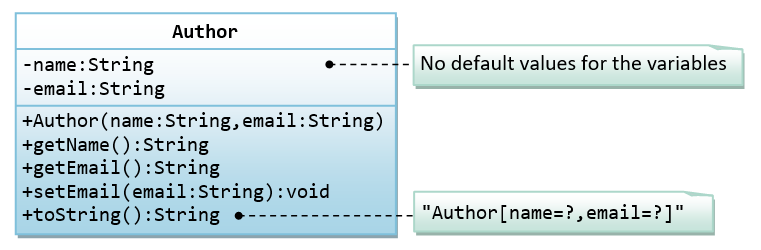Below is a test driver:

```public class TestMain {
public static void main(String[] args) {
// Test Author class
Author a1 = new Author("Tan Ah Teck", "ahteck@nowhere.com");
System.out.println(a1);

a1.setEmail("ahteck@somewhere.com");
System.out.println(a1);
System.out.println("name is: " + a1.getName());
System.out.println("email is: " + a1.getEmail());

// Test Book class
Book b1 = new Book("12345", "Java for dummies", a1, 8.8, 88);
System.out.println(b1);

b1.setPrice(9.9);
b1.setQty(99);
System.out.println(b1);
System.out.println("isbn is: " + b1.getIsbn());
System.out.println("name is: " + b1.getName());
System.out.println("price is: " + b1.getPrice());
System.out.println("qty is: " + b1.getQty());
System.out.println("author is: " + b1.getAuthor());  // Author's toString()
System.out.println("author's name: " + b1.getAuthorName());
System.out.println("author's name: " + b1.getAuthor().getName());
System.out.println("author's email: " + b1.getAuthor().getEmail());
}
}```

The expected output is:

```Author[name=Tan Ah Teck,email=ahteck@nowhere.com]
Author[name=Tan Ah Teck,email=ahteck@somewhere.com]
name is: Tan Ah Teck
email is: ahteck@somewhere.com
Book[isbn=12345,name=Java for dummies,Author[name=Tan Ah Teck,email=ahteck@somewhere.com],price=8.8,qty=88]
Book[isbn=12345,name=Java for dummies,Author[name=Tan Ah Teck,email=ahteck@somewhere.com],price=9.9,qty=99]
isbn is: Java for dummies
name is: Java for dummies
price is: 9.9
qty is: 99
author is: Author[name=Tan Ah Teck,email=ahteck@somewhere.com]
author's name: Tan Ah Teck
author's name: Tan Ah Teck
author's email: ahteck@somewhere.com```

#### Ex: The Customer and Invoice classes

A class called `Customer`, which models a customer in a transaction, is designed as shown in the class diagram. A class called `Invoice`, which models an invoice for a particular customer and composes an instance of `Customer` as its instance variable, is also shown. Write the `Customer` and `Invoice` classes.Below is a test driver:

```public class TestMain {
public static void main(String[] args) {
// Test Customer class
Customer c1 = new Customer(88, "Tan Ah Teck", 10);
System.out.println(c1);  // Customer's toString()

c1.setDiscount(8);
System.out.println(c1);
System.out.println("id is: " + c1.getId());
System.out.println("name is: " + c1.getName());
System.out.println("discount is: " + c1.getDiscount());

// Test Invoice class
Invoice inv1 = new Invoice(101, c1, 888.8);
System.out.println(inv1);

inv1.setAmount(999.9);
System.out.println(inv1);
System.out.println("id is: " + inv1.getId());
System.out.println("customer is: " + inv1.getCustomer());  // Customer's toString()
System.out.println("amount is: " + inv1.getAmount());
System.out.println("customer's id is: " + inv1.getCustomerId());
System.out.println("customer's name is: " + inv1.getCustomerName());
System.out.println("customer's discount is: " + inv1.getCustomerDiscount());
System.out.printf("amount after discount is: %.2f%n", inv1.getAmountAfterDiscount());
}
}```

The expected output is:

```Tan Ah Teck(88)(10%)
Tan Ah Teck(88)(8%)
id is: 88
name is: Tan Ah Teck
discount is: 8
Invoice[id=101,customer=Tan Ah Teck(88)(8%),amount=888.8]
Invoice[id=101,customer=Tan Ah Teck(88)(8%),amount=999.9]
id is: 101
customer is: Tan Ah Teck(88)(8%)
amount is: 999.9
customer's id is: 88
customer's name is: Tan Ah Teck
customer's discount is: 8
amount after discount is: 919.91```

#### Ex: The Customer and Account classes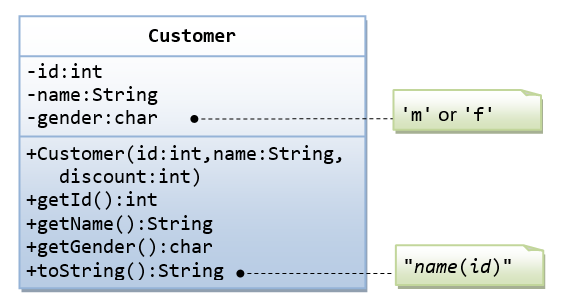The `Customer` class models a customer is design as shown in the class diagram. Write the codes for the `Customer` class and a test driver to test all the `public` methods.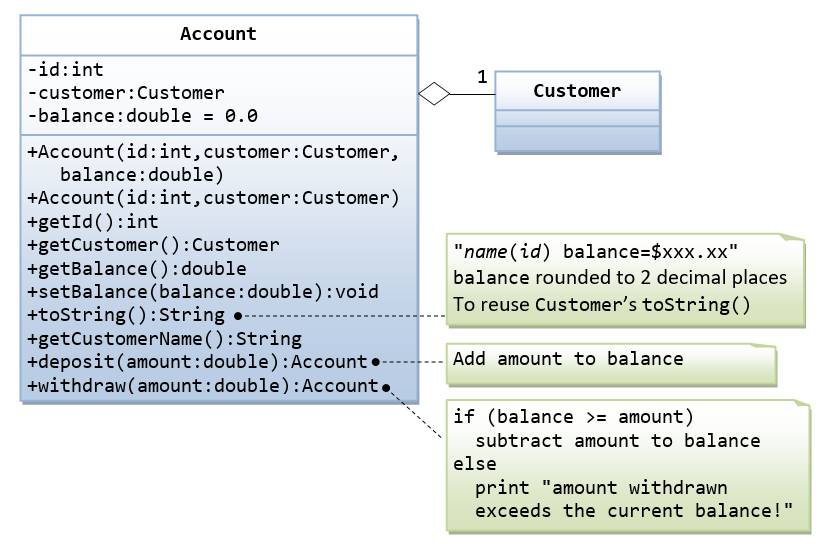The `Account` class models a bank account, design as shown in the class diagram, composes a `Customer` instance (written earlier) as its member. Write the codes for the `Account` class and a test driver to test all the `public` methods.

#### Ex: The MyPoint Class

A class called `MyPoint`, which models a 2D point with x and y coordinates, is designed as shown in the class diagram.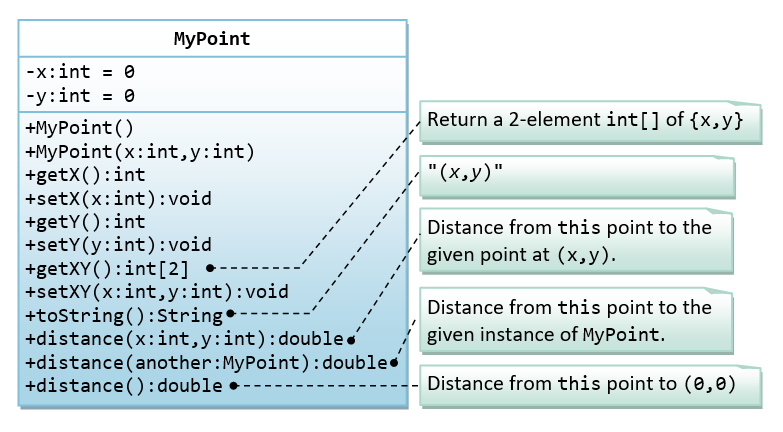It contains:

• Two instance variables `x` (`int`) and `y` (`int`).
• A default (or "no-argument" or "no-arg") constructor that construct a point at the default location of `(0, 0)`.
• A overloaded constructor that constructs a point with the given `x` and `y` coordinates.
• Getter and setter for the instance variables `x` and `y`.
• A method `setXY()` to set both `x` and `y`.
• A method `getXY()` which returns the x and y in a 2-element `int` array.
• A `toString()` method that returns a string description of the instance in the format "`(x, y)`".
• A method called `distance(int x, int y)` that returns the distance from this point to another point at the given `(x, y)` coordinates, e.g.,
```MyPoint p1 = new MyPoint(3, 4);
System.out.println(p1.distance(5, 6));```
• An overloaded `distance(MyPoint another)` that returns the distance from this point to the given `MyPoint` instance (called `another`), e.g.,
```MyPoint p1 = new MyPoint(3, 4);
MyPoint p2 = new MyPoint(5, 6);
System.out.println(p1.distance(p2));```
• Another overloaded `distance()` method that returns the distance from `this` point to the origin `(0,0)`, e.g.,
```MyPoint p1 = new MyPoint(3, 4);
System.out.println(p1.distance());```

You are required to:

1. Write the code for the class `MyPoint`. Also write a test program (called `TestMyPoint`) to test all the methods defined in the class.
Hints:
```// Overloading method distance()
// This version takes two ints as arguments
public double distance(int x, int y) {
int xDiff = this.x – x;
int yDiff = ......
return Math.sqrt(xDiff*xDiff + yDiff*yDiff);
}

// This version takes a MyPoint instance as argument
public double distance(MyPoint another) {
int xDiff = this.x – another.x;
.......
}```
```// Test program to test all constructors and public methods
MyPoint p1 = new MyPoint();  // Test constructor
System.out.println(p1);      // Test toString()
p1.setX(8);   // Test setters
p1.setY(6);
System.out.println("x is: " + p1.getX());  // Test getters
System.out.println("y is: " + p1.getY());
p1.setXY(3, 0);   // Test setXY()
System.out.println(p1.getXY());  // Test getXY()
System.out.println(p1.getXY());
System.out.println(p1);

MyPoint p2 = new MyPoint(0, 4);  // Test another constructor
System.out.println(p2);
// Testing the overloaded methods distance()
System.out.println(p1.distance(p2));    // which version?
System.out.println(p2.distance(p1));    // which version?
System.out.println(p1.distance(5, 6));  // which version?
System.out.println(p1.distance());      // which version?
```
2. Write a program that allocates `10` points in an array of `MyPoint`, and initializes to `(1, 1)`, `(2, 2)`, ... `(10, 10)`.
Hints: You need to allocate the array, as well as each of the 10 `MyPoint` instances.  In other words, you need to issue 11 `new`, 1 for the array and 10 for the `MyPoint` instances.
```MyPoint[] points = new MyPoint;  // Declare and allocate an array of MyPoint
for (int i = 0; i < points.length; i++) {
points[i] = new MyPoint(...);    // Allocate each of MyPoint instances
}
// use a loop to print all the points
```

Notes: Point is such a common entity that JDK certainly provided for in all flavors.

#### Ex: The MyLine and MyPoint Classes

A class called `MyLine`, which models a line with a begin point at (x1, y1) and an end point at (x2, y2), is designed as shown in the class diagram. The `MyLine` class uses two `MyPoint` instances (written in the earlier exercise) as its begin and end points. Write the `MyLine` class. Also write a test driver to test all the public methods in the `MyLine` class.#### Ex: The MyCircle and MyPoint Classes

A class called `MyCircle`, which models a circle with a center and a radius, is designed as shown in the class diagram. The `MyCircle` class uses a `MyPoint` instance (written in the earlier exercise) as its center.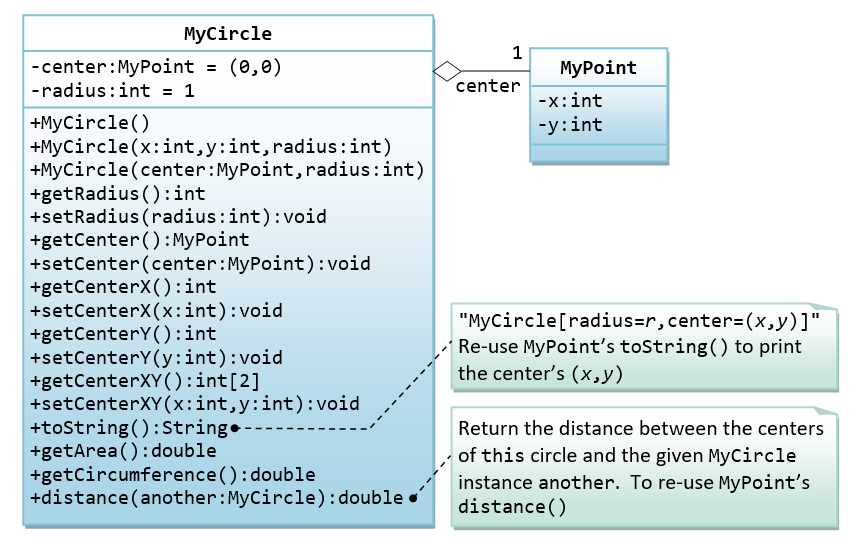The class contains:

• Two `private` instance variables: `center` (an instance of `MyPoint`) and `radius` (`int`).
• A constructor that constructs a circle with the given center's (`x`, `y`) and `radius`.
• An overloaded constructor that constructs a `MyCircle` given a `MyPoint` instance as `center`, and `radius`.
• A default constructor that construct a circle with center at `(0,0)` and radius of `1`.
• Various getters and setters.
• A `toString()` method that returns a string description of this instance in the format "`MyCircle[radius=r,center=(x,y)]`". You shall reuse the `toString()` of `MyPoint`.
• `getArea()` and `getCircumference()` methods that return the area and circumference of `this` circle in `double`.
• A `distance(MyCircle another)` method that returns the distance of the centers from `this` instance and the given `MyCircle` instance.  You should use `MyPoint`’s `distance()` method to compute this distance.

Write the `MyCircle` class. Also write a test driver (called `TestMyCircle`) to test all the public methods defined in the class.

Hints:

```// Constructors
public MyCircle(int x, int y, int radius) {
// Need to construct an instance of MyPoint for the variable center
center = new MyPoint(x, y);
}
public MyCircle(MyPoint center, int radius) {
// An instance of MyPoint already constructed by caller; simply assign.
this.center = center;
......
}
public MyCircle() {
center = new MyPoint(.....);  // construct MyPoint instance
}

// Returns the x-coordinate of the center of this MyCircle
public int getCenterX() {
return center.getX();   // cannot use center.x and x is private in MyPoint
}

// Returns the distance of the center for this MyCircle and another MyCircle
public double distance(MyCircle another) {
return center.distance(another.center); // use distance() of MyPoint
}```

#### Ex: The MyTriangle and MyPoint Classes

A class called `MyTriangle`, which models a triangle with 3 vertices, is designed as shown in the class diagram. The `MyTriangle` class uses three `MyPoint` instances (created in the earlier exercise) as the three vertices.It contains:

• Three `private` instance variables `v1`, `v2`, `v3` (instances of `MyPoint`), for the three vertices.
• A constructor that constructs a `MyTriangle` with three set of coordinates, `v1=(x1, y1)`, `v2=(x2, y2)`, `v3=(x3, y3)`.
• An overloaded constructor that constructs a `MyTriangle` given three instances of `MyPoint`.
• A `toString()` method that returns a string description of the instance in the format "`MyTriangle[v1=(x1,y1),v2=(x2,y2),v3=(x3,y3)]`".
• A `getPerimeter()` method that returns the length of the perimeter in double. You should use the `distance()` method of `MyPoint` to compute the perimeter.
• A method `printType()`, which prints "`equilateral`" if all the three sides are equal, "`isosceles`" if any two of the three sides are equal, or "`scalene`" if the three sides are different.

Write the `MyTriangle` class. Also write a test driver (called `TestMyTriangle`) to test all the `public` methods defined in the class.

#### Ex: The MyRectangle and MyPoint Classes

Design a `MyRectangle` class which is composed of two `MyPoint` instances as its top-left and bottom-right corners. Draw the class diagrams, write the codes, and write the test drivers.

### More Exercises on Classes

#### Ex: The MyComplex class

A class called `MyComplex`, which models a complex number with real and imaginary parts, is designed as shown in the class diagram.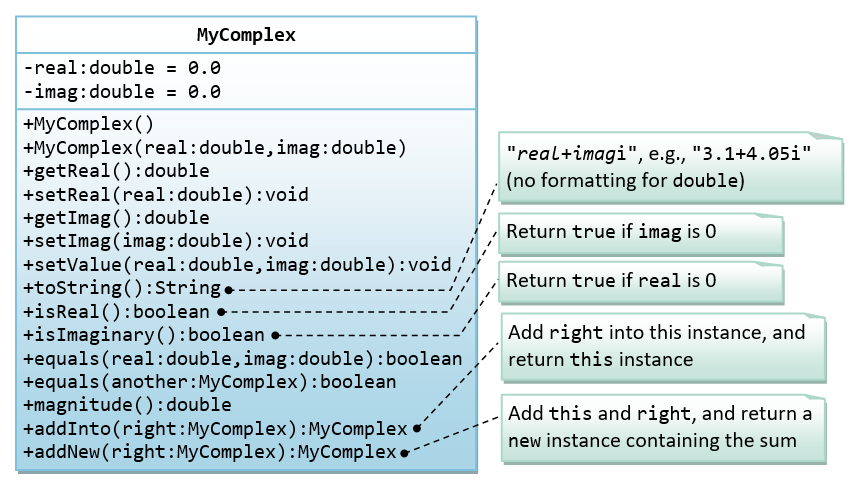It contains:

• Two instance variable named `real` (`double`) and `imag` (`double`) which stores the real and imaginary parts of the complex number, respectively.
• A constructor that creates a `MyComplex` instance with the given real and imaginary values.
• A default constructor that create a MyComplex at `0.0 + 0.0i`.
• Getters and setters for instance variables `real` and `imag`.
• A method `setValue()` to set the value of the complex number.
• A `toString()` that returns "`(x + yi)`" where `x` and `y` are the real and imaginary parts, respectively.
• Methods `isReal()` and `isImaginary()` that returns `true` if this complex number is real or imaginary, respectively.
Hints:
`return (imag == 0);`
• A method `equals(double real, double imag)` that returns `true` if `this` complex number is equal to the given complex number (real, imag).
Hints:
`return (this.real == real && this.imag == imag);`
• An overloaded `equals(MyComplex another)` that returns `true` if this complex number is equal to the given `MyComplex` instance `another`.
Hints:
`return (this.real == another.real && this.imag == another.imag);`
• A method `magnitude()` that returns the magnitude of this complex number.
`magnitude(x+yi) = Math.sqrt(x*x + y*y)`
• Methods `addInto(MyComplex right)` that adds and subtract the given `MyComplex` instance (called `right`) into `this` instance and returns `this` instance.
```(a + bi) + (c + di) = (a+c) + (b+d)i
```
Hints:
`return this;  // return "this" instance`
• Methods `addNew(MyComplex right)` that adds `this` instance with the given `MyComplex` instance called `right`, and returns a new `MyComplex` instance containing the result.
Hint:
```// construct a new instance and return the constructed instance
return new MyComplex(..., ...);```

You are required to:

1. Write the `MyComplex` class.
2. Write a test driver to test all the `public` methods defined in the class.
3. Write an application called `MyComplexApp` that uses the `MyComplex` class. The application shall prompt the user for two complex numbers, print their values, check for real, imaginary and equality, and carry out all the arithmetic operations.
```Enter complex number 1 (real and imaginary part): 1.1 2.2
Enter complex number 2 (real and imaginary part): 3.3 4.4

Number 1 is: (1.1 + 2.2i)
(1.1 + 2.2i) is NOT a pure real number
(1.1 + 2.2i) is NOT a pure imaginary number

Number 2 is: (3.3 + 4.4i)
(3.3 + 4.4i) is NOT a pure real number
(3.3 + 4.4i) is NOT a pure imaginary number

(1.1 + 2.2i) is NOT equal to (3.3 + 4.4i)
(1.1 + 2.2i) + (3.3 + 4.4i) = (4.4 + 6.6000000000000005i)```

Try: A (more) complete design of `MyComplex` class is shown below: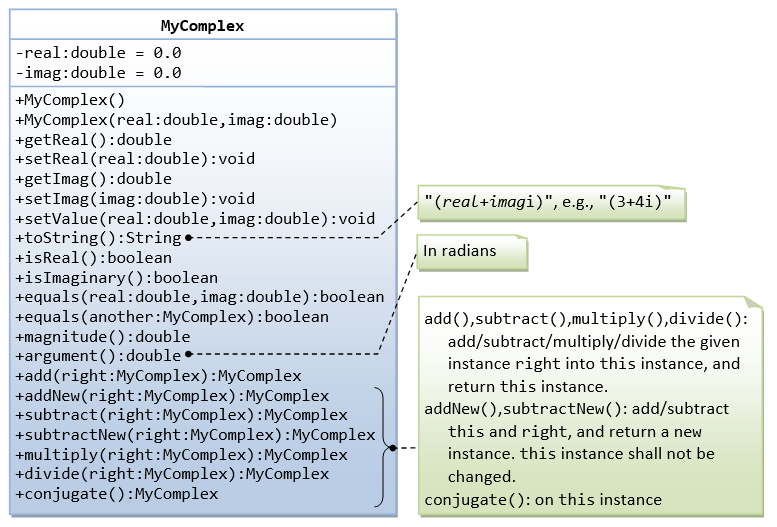• Methods `argument()` that returns the argument of this complex number in radians (`double`).
`arg(x+yi) = Math.atan2(y, x) (in radians)`
Note: The `Math` library has two arc-tangent methods, `Math.atan(double)` and `Math.atan2(double, double)`. We commonly use the `Math.atan2(y, x)` instead of `Math.atan(y/x)` to avoid division by zero. Read the documentation of `Math` class in package `java.lang`.
• The method `addInto()` is renamed `add()`. Also added `subtract()` and `subtractNew()`.
• Methods `multiply(MyComplex right)` and `divide(MyComplex right)` that multiplies and divides `this` instance with the given `MyComplex` instance `right`, and keeps the result in `this` instance, and returns this instance.
```(a + bi) * (c + di) = (ac - bd) + (ad + bc)i
(a + bi) / (c + di) = [(a + bi) * (c – di)] / (c*c + d*d)```
• A method `conjugate()` that operates on `this` instance and returns `this` instance containing the complex conjugate.
`conjugate(x+yi) = x - yi`

Take note that there are a few flaws in the design of this class, which was introduced solely for teaching purpose:

• Comparing `double`s in `equal()` using "`==`" may produce unexpected outcome. For example, `(2.2+4.4)==6.6` returns `false`. It is common to define a small threshold called `EPSILON` (set to about `10^-8`) for comparing floating point numbers.
• The method `addNew()`, `subtractNew()` produce new instances, whereas `add()`, `subtract()`, `multiply()`, `divide()` and `conjugate()` modify `this` instance. There is inconsistency in the design (introduced for teaching purpose).

Also take note that methods such as `add()` returns an instance of `MyComplex`. Hence, you can place the result inside a `System.out.println()` (which implicitly invoke the `toString()`). You can also chain the operations, e.g., `c1.add(c2).add(c3)` (same as `(c1.add(c2)).add(c3))`, or `c1.add(c2).subtract(c3)`.

#### Ex: The MyPolynomial Class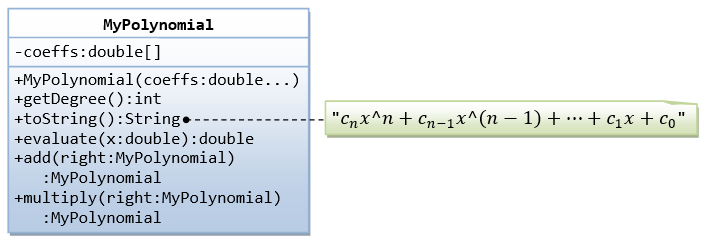A class called `MyPolynomial`, which models polynomials of degree-`n` (see equation), is designed as shown in the class diagram.It contains:

• An instance variable named `coeffs`, which stores the coefficients of the n-degree polynomial in a `double` array of size `n+1`, where c0 is kept at index 0.
• A constructor `MyPolynomial(coeffs:double...)` that takes a variable number of doubles to initialize the coeffs array, where the first argument corresponds to c0.
The three dots is known as varargs (variable number of arguments), which is a new feature introduced in JDK 1.5. It accepts an array or a sequence of comma-separated arguments. The compiler automatically packs the comma-separated arguments in an array. The three dots can only be used for the last argument of the method.
Hints:
```public class MyPolynomial {
private double[] coeffs;
public MyPolynomial(double... coeffs) {  // varargs
this.coeffs = coeffs;                 // varargs is treated as array
}
......
}

// Test program
// Can invoke with a variable number of arguments
MyPolynomial p1 = new MyPolynomial(1.1, 2.2, 3.3);
MyPolynomial p1 = new MyPolynomial(1.1, 2.2, 3.3, 4.4, 5.5);
// Can also invoke with an array
Double coeffs = {1.2, 3.4, 5.6, 7.8}
MyPolynomial p2 = new MyPolynomial(coeffs);```
• A method `getDegree()` that returns the degree of this polynomial.
• A method `toString()` that returns "cnx^n+cn-1x^(n-1)+...+c1x+c0".
• A method `evaluate(double x)` that evaluate the polynomial for the given `x`, by substituting the given `x` into the polynomial expression.
• Methods `add()` and `multiply()` that adds and multiplies this polynomial with the given `MyPolynomial` instance `another`, and returns `this` instance that contains the result.

Write the `MyPolynomial` class. Also write a test driver (called `TestMyPolynomial`) to test all the `public` methods defined in the class.

Question: Do you need to keep the degree of the polynomial as an instance variable in the `MyPolynomial` class in Java? How about C/C++? Why?

#### Ex: Using JDK's BigInteger Class

Recall that primitive integer type `byte`, `short`, `int` and `long` represent 8-, 16-, 32-, and 64-bit signed integers, respectively. You cannot use them for integers bigger than 64 bits. Java API provides a class called `BigInteger` in a package called `java.math`. Study the API of the `BigInteger` class (Java API ⇒ From "Packages", choose "java.math" " From "classes", choose "BigInteger" " Study the constructors (choose "CONSTR") on how to construct a `BigInteger` instance, and the public methods available (choose "METHOD"). Look for methods for adding and multiplying two `BigIntegers`.

Write a program called `TestBigInteger` that:

1. adds "11111111111111111111111111111111111111111111111111111111111111" to "22222222222222222222222222222222222222222222222222" and prints the result.
2. multiplies the above two number and prints the result.

Hints:

```import java.math.BigInteger
public class TestBigInteger {
public static void main(String[] args) {
BigInteger i1 = new BigInteger(...);
BigInteger i2 = new BigInteger(...);
.......
}
}```

#### Ex: The MyTime Class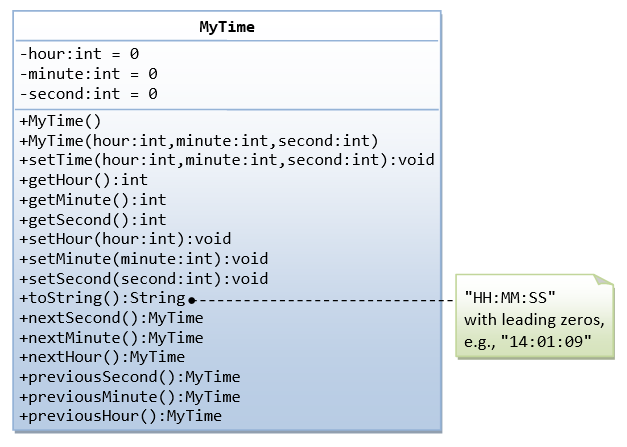A class called `MyTime`, which models a time instance, is designed as shown in the class diagram.

It contains the following `private` instance variables:

• `hour`: between 0 to 23.
• `minute`: between 0 to 59.
• `Second`: between 0 to 59.

You are required to perform input validation.

It contains the following `public` methods:

• `setTime(int hour, int minute, int second)`: It shall check if the given `hour`, `minute` and `second` are valid before setting the instance variables.
(Advanced: Otherwise, it shall throw an `IllegalArgumentException` with the message "Invalid hour, minute, or second!".)
• Setters `setHour(int hour)`, `setMinute(int minute)`, `setSecond(int second)`: It shall check if the parameters are valid, similar to the above.
• Getters `getHour()`, `getMinute()`, `getSecond()`.
• `toString()`: returns "`HH:MM:SS`".
• `nextSecond()`: Update this instance to the next second and return this instance. Take note that the `nextSecond()` of `23:59:59` is `00:00:00`.
• `nextMinute()`, `nextHour()`, `previousSecond()`, `previousMinute()`, `previousHour()`: similar to the above.

Write the code for the `MyTime` class. Also write a test driver (called `TestMyTime`) to test all the `public` methods defined in the `MyTime` class.

#### Ex: The MyDate ClassA class called `MyDate`, which models a date instance, is defined as shown in the class diagram.

The `MyDate` class contains the following `private` instance variables:

• `year` (`int`): Between `1` to `9999`.
• `month` (`int`): Between `1` (Jan) to `12` (Dec).
• `day` (`int`): Between `1` to `28|29|30|31`, where the last day depends on the month and whether it is a leap year for Feb (`28|29`).

It also contains the following `public` `static final ` variables (drawn with underlined in the class diagram):

• `MONTHS` (`String[]`), `DAYS` (`String[]`), and DAY_IN_`MONTHS` (`int[]`): `static` variables, initialized as shown, which are used in the methods.

The `MyDate` class has the following `public` `static` methods (drawn with underlined in the class diagram):

• `isLeapYear(int year)`: returns `true` if the given `year` is a leap year. A year is a leap year if it is divisible by 4 but not by 100, or it is divisible by 400.
• `isValidDate(int year, int month, int day)`: returns `true` if the given `year`, `month`, and `day` constitute a valid date. Assume that `year` is between `1` and `9999`, `month` is between `1` (Jan) to `12` (Dec) and `day` shall be between `1` and `28|29|30|31` depending on the `month` and whether it is a leap year on Feb.
• `getDayOfWeek(int year, int month, int day)`: returns the day of the week, where `0` for Sun, `1` for Mon, ..., `6` for Sat, for the given date. Assume that the date is valid. Read the earlier exercise on how to determine the day of the week (or Wiki "Determination of the day of the week").

The `MyDate` class has one constructor, which takes 3 parameters: `year`, `month` and `day`. It shall invoke `setDate()` method (to be described later) to set the instance variables.

The `MyDate` class has the following `public` methods:

• `setDate(int year, int month, int day)`: It shall invoke the `static` method `isValidDate()` to verify that the given `year`, `month` and `day` constitute a valid date.
(Advanced: Otherwise, it shall throw an `IllegalArgumentException` with the message "Invalid year, month, or day!".)
• `setYear(int year)`: It shall verify that the given `year` is between `1` and `9999`.
(Advanced: Otherwise, it shall throw an `IllegalArgumentException` with the message "Invalid year!".)
• `setMonth(int month)`: It shall verify that the given `month` is between `1` and `12`.
(Advanced: Otherwise, it shall throw an `IllegalArgumentException` with the message "Invalid month!".)
• `setDay(int day)`: It shall verify that the given `day` is between `1` and `dayMax`, where `dayMax` depends on the `month` and whether it is a leap year for Feb.
(Advanced: Otherwise, it shall throw an `IllegalArgumentException` with the message "Invalid month!".)
• `getYear()`, `getMonth()`, `getDay()`: return the value for the `year`, `month` and `day`, respectively.
• `toString()`: returns a date string in the format "`xxxday d mmm yyyy`", e.g., "Tuesday 14 Feb 2012".
• `nextDay()`: update `this` instance to the next day and return `this` instance. Take note that `nextDay()` for `31 Dec 2000` shall be `1 Jan 2001`.
• `nextMonth()`: update `this` instance to the next month and return `this` instance. Take note that `nextMonth()` for `31 Oct 2012` shall be `30 Nov 2012`.
• `nextYear()`: update `this` instance to the next year and return `this` instance. Take note that `nextYear()` for `29 Feb 2012` shall be `28 Feb 2013`.
(Advanced: throw an `IllegalStateException` with the message "Year out of range!" if year > 9999.)
• `previousDay()`, `previousMonth()`, `previousYear()`: similar to the above.

Write the code for the `MyDate` class.

Use the following test statements to test the `MyDate` class:

```MyDate d1 = new MyDate(2012, 2, 28);
System.out.println(d1);             // Tuesday 28 Feb 2012
System.out.println(d1.nextDay());   // Wednesday 29 Feb 2012
System.out.println(d1.nextDay());   // Thursday 1 Mar 2012
System.out.println(d1.nextMonth()); // Sunday 1 Apr 2012
System.out.println(d1.nextYear());  // Monday 1 Apr 2013

MyDate d2 = new MyDate(2012, 1, 2);
System.out.println(d2);                 // Monday 2 Jan 2012
System.out.println(d2.previousDay());   // Sunday 1 Jan 2012
System.out.println(d2.previousDay());   // Saturday 31 Dec 2011
System.out.println(d2.previousMonth()); // Wednesday 30 Nov 2011
System.out.println(d2.previousYear());  // Tuesday 30 Nov 2010

MyDate d3 = new MyDate(2012, 2, 29);
System.out.println(d3.previousYear());  // Monday 28 Feb 2011

// MyDate d4 = new MyDate(2099, 11, 31); // Invalid year, month, or day!
// MyDate d5 = new MyDate(2011, 2, 29);  // Invalid year, month, or day!```

Write a test program that tests the `nextDay()` in a loop, by printing the dates from `28 Dec 2011` to `2 Mar 2012`.

#### Ex: Bouncing Balls - Ball and Container ClassesA class called `Ball` is designed as shown in the class diagram.

The `Ball` class contains the following `private` instance variables:

• `x`, `y` and `radius`, which represent the ball's center `(x, y)` co-ordinates and the radius, respectively.
• `xDelta` (`Δx`) and `yDelta` (`Δy`), which represent the displacement (movement) per step, in the `x` and `y` direction respectively.

The `Ball` class contains the following `public` methods:

• A constructor which accepts `x`, `y`, `radius`, `speed`, and `direction` as arguments. For user friendliness, user specifies `speed` (in pixels per step) and `direction` (in degrees in the range of `(-180°, 180°]`). For the internal operations, the `speed` and `direction` are to be converted to `(Δx, Δy)` in the internal representation. Note that the y-axis of the Java graphics coordinate system is inverted, i.e., the origin `(0, 0)` is located at the top-left corner.```Δx = d × cos(θ)
Δy = -d × sin(θ)```
• Getter and setter for all the instance variables.
• A method `move()` which move the ball by one step.
```x += Δx
y += Δy```
• `reflectHorizontal()` which reflects the ball horizontally (i.e., hitting a vertical wall)
```Δx = -Δx
Δy no changes```
• `reflectVertical()` (the ball hits a horizontal wall).
```Δx no changes
Δy = -Δy```
• `toString()` which prints the message "`Ball at (x, y) of velocity (Δx, Δy)`".

Write the `Ball` class. Also write a test program to test all the methods defined in the class.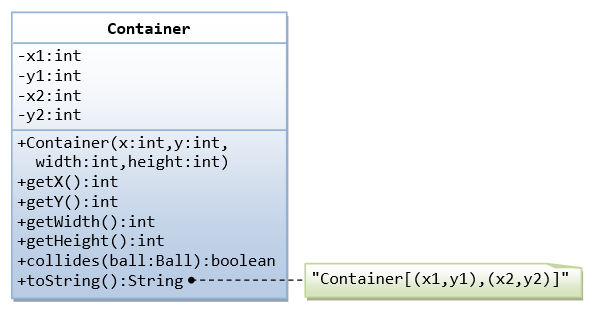A class called `Container`, which represents the enclosing box for the ball, is designed as shown in the class diagram. It contains:

• Instance variables `(x1, y1)` and `(x2, y2)` which denote the top-left and bottom-right corners of the rectangular box.
• A constructor which accepts `(x, y)` of the top-left corner, `width` and `height` as argument, and converts them into the internal representation (i.e., `x2=x1+width-1`). `Width` and `height` is used in the argument for safer operation (there is no need to check the validity of `x2>x1` etc.).
• A `toString()` method that returns "`Container at (x1,y1) to (x2, y2)`".
• A `boolean` method called `collidesWith(Ball)`, which check if the given `Ball` is outside the bounds of the container box. If so, it invokes the `Ball`'s `reflectHorizontal()` and/or `reflectVertical()` to change the movement direction of the ball, and returns `true`.
```public boolean collidesWith(Ball ball) {
if (ball.getX() - ball.getRadius() <= this.x1 ||
ball.getX() - ball.getRadius() >= this.x2) {
ball.reflectHorizontal();
return true;
}
......
}```

Use the following statements to test your program:

```Ball ball = new Ball(50, 50, 5, 10, 30);
Container box = new Container(0, 0, 100, 100);
for (int step = 0; step < 100; ++step) {
ball.move();
box.collidesWith(ball);
System.out.println(ball); // manual check the position of the ball
}```

#### Ex: The Ball and Player ClassesThe `Ball` class, which models the ball in a soccer game, is designed as shown in the class diagram. Write the codes for the `Ball` class and a test driver to test all the `public` methods.The `Player` class, which models the players in a soccer game, is designed as shown in the class diagram. The Player interacts with the Ball (written earlier). Write the codes for the `Player` class and a test driver to test all the `public` methods. Make your assumption for the `kick()`.

Can you write a very simple soccer game with 2 teams of players and a ball, inside a soccer field?

### Exercises on Inheritance

#### An Introduction to OOP Inheritance by Example - The Circle and Cylinder Classes

This exercise shall guide you through the important concepts in inheritance.In this exercise, a subclass called `Cylinder` is derived from the superclass `Circle` as shown in the class diagram (where an an arrow pointing up from the subclass to its superclass). Study how the subclass `Cylinder` invokes the superclass' constructors (via `super()` and `super(radius)`) and inherits the variables and methods from the superclass `Circle`.

You can reuse the `Circle` class that you have created in the previous exercise. Make sure that you keep "`Circle.class`" in the same directory.

```public class Cylinder extends Circle {  // Save as "Cylinder.java"
private double height;  // private variable

// Constructor with default color, radius and height
public Cylinder() {
super();        // call superclass no-arg constructor Circle()
height = 1.0;
}
// Constructor with default radius, color but given height
public Cylinder(double height) {
super();        // call superclass no-arg constructor Circle()
this.height = height;
}
// Constructor with default color, but given radius, height
public Cylinder(double radius, double height) {
super(radius);  // call superclass constructor Circle(r)
this.height = height;
}

// A public method for retrieving the height
public double getHeight() {
return height;
}

// A public method for computing the volume of cylinder
//  use superclass method getArea() to get the base area
public double getVolume() {
return getArea()*height;
}
}```

Write a test program (says `TestCylinder`) to test the `Cylinder` class created, as follow:

```public class TestCylinder {  // save as "TestCylinder.java"
public static void main (String[] args) {
// Declare and allocate a new instance of cylinder
//   with default color, radius, and height
Cylinder c1 = new Cylinder();
System.out.println("Cylinder:"
+ " height=" + c1.getHeight()
+ " base area=" + c1.getArea()
+ " volume=" + c1.getVolume());

// Declare and allocate a new instance of cylinder
//   specifying height, with default color and radius
Cylinder c2 = new Cylinder(10.0);
System.out.println("Cylinder:"
+ " height=" + c2.getHeight()
+ " base area=" + c2.getArea()
+ " volume=" + c2.getVolume());

// Declare and allocate a new instance of cylinder
//   specifying radius and height, with default color
Cylinder c3 = new Cylinder(2.0, 10.0);
System.out.println("Cylinder:"
+ " height=" + c3.getHeight()
+ " base area=" + c3.getArea()
+ " volume=" + c3.getVolume());
}
}```

Method Overriding and "Super": The subclass `Cylinder` inherits `getArea()` method from its superclass Circle. Try overriding the `getArea()` method in the subclass `Cylinder` to compute the surface area (=2π×radius×height + 2×base-area) of the cylinder instead of base area. That is, if `getArea()` is called by a `Circle` instance, it returns the area. If `getArea()` is called by a `Cylinder` instance, it returns the surface area of the cylinder.

If you override the `getArea()` in the subclass `Cylinder`, the `getVolume()` no longer works. This is because the `getVolume()` uses the overridden `getArea()` method found in the same class. (Java runtime will search the superclass only if it cannot locate the method in this class). Fix the `getVolume()`.

Hints: After overridding the `getArea()` in subclass `Cylinder`, you can choose to invoke the `getArea()` of the superclass `Circle` by calling `super.getArea()`.

TRY:

Provide a `toString()` method to the `Cylinder` class, which overrides the `toString()` inherited from the superclass `Circle`, e.g.,

```@Override
public String toString() {      // in Cylinder class
return "Cylinder: subclass of " + super.toString()  // use Circle's toString()
+ " height=" + height;
}```

Try out the `toString()` method in `TestCylinder`.

Note: `@Override` is known as annotation (introduced in JDK 1.5), which asks compiler to check whether there is such a method in the superclass to be overridden. This helps greatly if you misspell the name of the `toString()`. If `@Override` is not used and `toString()` is misspelled as `ToString()`, it will be treated as a new method in the subclass, instead of overriding the superclass. If `@Override` is used, the compiler will signal an error. `@Override` annotation is optional, but certainly nice to have.

#### Ex: Superclass Person and its subclasses

Write the classes as shown in the following class diagram. Mark all the overridden methods with annotation `@Override`.#### Ex: Point2D and Point3D

Write the classes as shown in the following class diagram. Mark all the overridden methods with annotation `@Override`.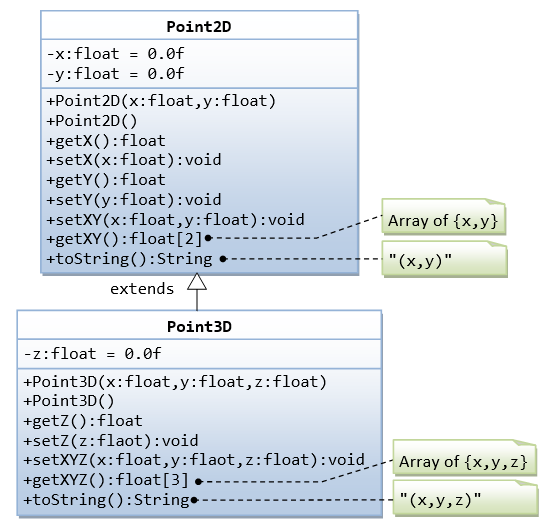##### Hints:
1. You cannot assign floating-point literal say `1.1` (which is a `double`) to a `float` variable, you need to add a suffix f, e.g. `0.0f`, `1.1f`.
2. The instance variables `x` and `y` are `private` in `Point2D` and cannot be accessed directly in the subclass `Point3D`. You need to access via the `public` getters and setters. For example,
```    public void setXYZ(float x, float y, float z) {
setX(x);     // or super.setX(x), use setter in superclass
setY(y);
this.z = z;
}```
3. The method `getXY()` shall return a `float` array:
```	public float[] getXY() {
float[] result = new float;  // construct an array of 2 elements
result = ...
result = ...
return result;  // return the array
}```

#### Ex: Point and MovablePoint

Write the classes as shown in the following class diagram. Mark all the overridden methods with annotation `@Override.`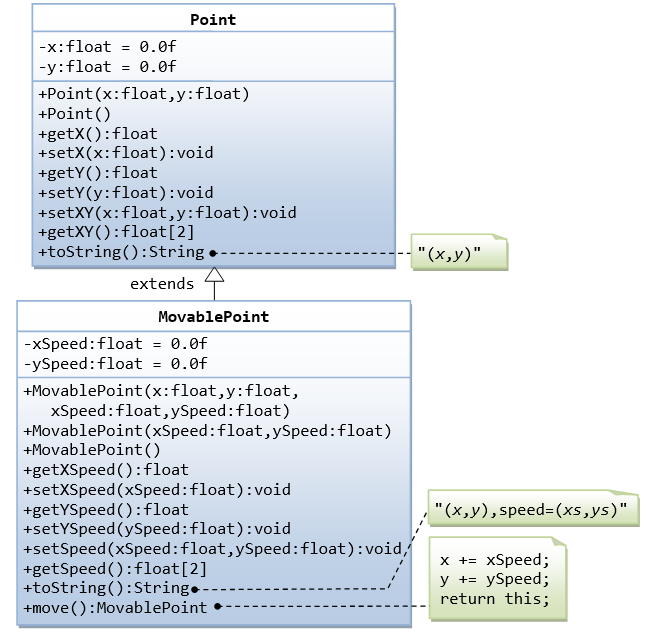##### Hints
1. You cannot assign floating-point literal say `1.1` (which is a `double`) to a `float` variable, you need to add a suffix f, e.g. `0.0f`, `1.1f`.
2. The instance variables `x` and `y` are `private` in `Point` and cannot be accessed directly in the subclass `MovablePoint`. You need to access via the `public` getters and setters. For example, you cannot write `x += xSpeed`, you need to write `setX(getX() + xSpeed)`.

#### Ex: Superclass Shape and its subclasses Circle, Rectangle and SquareWrite a superclass called `Shape` (as shown in the class diagram), which contains:

• Two instance variables `color` (`String`) and `filled` (`boolean`).
• Two constructors: a no-arg (no-argument) constructor that initializes the `color` to "green" and `filled` to `true`, and a constructor that initializes the `color` and `filled` to the given values.
• Getter and setter for all the instance variables. By convention, the getter for a `boolean` variable `xxx` is called `isXXX()` (instead of `getXxx()` for all the other types).
• A `toString()` method that returns "`A Shape with color of xxx and filled/Not filled`".

Write a test program to test all the methods defined in `Shape`.

Write two subclasses of `Shape` called `Circle` and `Rectangle`, as shown in the class diagram.

The `Circle` class contains:

• An instance variable `radius` (`double`).
• Three constructors as shown. The no-arg constructor initializes the radius to `1.0`.
• Getter and setter for the instance variable `radius`.
• Methods `getArea()` and `getPerimeter()`.
• Override the `toString()` method inherited, to return "`A Circle with radius=xxx, which is a subclass of yyy`", where `yyy` is the output of the `toString()` method from the superclass.

The `Rectangle` class contains:

• Two instance variables `width` (`double`) and `length` (`double`).
• Three constructors as shown. The no-arg constructor initializes the `width` and `length` to `1.0`.
• Getter and setter for all the instance variables.
• Methods `getArea()` and `getPerimeter()`.
• Override the `toString()` method inherited, to return "`A Rectangle with width=xxx and length=zzz, which is a subclass of yyy`", where `yyy` is the output of the `toString()` method from the superclass.

Write a class called `Square`, as a subclass of `Rectangle`. Convince yourself that `Square` can be modeled as a subclass of `Rectangle`. `Square` has no instance variable, but inherits the instance variables width and length from its superclass Rectangle.

• Provide the appropriate constructors (as shown in the class diagram). Hint:
```public Square(double side) {
super(side, side);  // Call superclass Rectangle(double, double)
}```
• Override the `toString()` method to return "`A Square with side=xxx, which is a subclass of yyy`", where `yyy` is the output of the `toString()` method from the superclass.
• Do you need to override the `getArea()` and `getPerimeter()`? Try them out.
• Override the `setLength()` and `setWidth()` to change both the `width` and `length`, so as to maintain the square geometry.

#### Ex: Superclass Animal and its subclasses

Write the codes for all the classes as shown in the class diagram.### Exercises on Composition vs Inheritance

They are two ways to reuse a class in your applications: composition and inheritance.

#### Ex: The Point and Line Classes

Let us begin with composition with the statement "a line composes of two points".

Complete the definition of the following two classes: `Point` and `Line`. The class `Line` composes 2 instances of class `Point`, representing the beginning and ending points of the line. Also write test classes for `Point` and `Line` (says `TestPoint` and `TestLine`).

```public class Point {
// Private variables
private int x;    // x co-ordinate
private int y;    // y co-ordinate

// Constructor
public Point (int x, int y) {......}

// Public methods
public String toString() {
return "Point: (" + x + "," + y + ")";
}

public int getX() {......}
public int getY() {......}
public void setX(int x) {......}
public void setY(int y) {......}
public void setXY(int x, int y) {......}
}```
```public class TestPoint {
public static void main(String[] args) {
Point p1 = new Point(10, 20);   // Construct a Point
System.out.println(p1);
// Try setting p1 to (100, 10).
......
}
}```
```public class Line {
// A line composes of two points (as instance variables)
private Point begin;    // beginning point
private Point end;      // ending point

// Constructors
public Line (Point begin, Point end) {  // caller to construct the Points
this.begin = begin;
......
}
public Line (int beginX, int beginY, int endX, int endY) {
begin = new Point(beginX, beginY);   // construct the Points here
......
}

// Public methods
public String toString() { ...... }

public Point getBegin() { ...... }
public Point getEnd() { ...... }
public void setBegin(......) { ...... }
public void setEnd(......) { ...... }

public int getBeginX() { ...... }
public int getBeginY() { ...... }
public int getEndX() { ...... }
public int getEndY() { ...... }

public void setBeginX(......) { ...... }
public void setBeginY(......) { ...... }
public void setBeginXY(......) { ...... }
public void setEndX(......) { ...... }
public void setEndY(......) { ...... }
public void setEndXY(......) { ...... }

public int getLength() { ...... } // Length of the line
// Math.sqrt(xDiff*xDiff + yDiff*yDiff)
// Math.atan2(yDiff, xDiff)
}```
```public class TestLine {
public static void main(String[] args) {
Line l1 = new Line(0, 0, 3, 4);
System.out.println(l1);

Point p1 = new Point(...);
Point p2 = new Point(...);
Line l2 = new Line(p1, p2);
System.out.println(l2);
...
}
}```

The class diagram for composition is as follows (where a diamond-hollow-head arrow pointing to its constituents):Instead of composition, we can design a `Line` class using `inheritance`. Instead of "a line composes of two points", we can say that "a line is a point extended by another point", as shown in the following class diagram: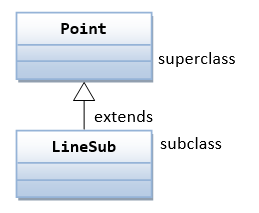Let's re-design the `Line` class (called `LineSub`) as a subclass of class `Point`. `LineSub` inherits the starting point from its superclass `Point`, and adds an ending point. Complete the class definition. Write a testing class called `TestLineSub` to test `LineSub`.

```public class LineSub extends Point {
// A line needs two points: begin and end.
// The begin point is inherited from its superclass Point.
// Private variables
Point end;               // Ending point

// Constructors
public LineSub (int beginX, int beginY, int endX, int endY) {
super(beginX, beginY);             // construct the begin Point
this.end = new Point(endX, endY);  // construct the end Point
}
public LineSub (Point begin, Point end) {  // caller to construct the Points
super(begin.getX(), begin.getY());      // need to reconstruct the begin Point
this.end = end;
}

// Public methods
// Inherits methods getX() and getY() from superclass Point
public String toString() { ... }

public Point getBegin() { ... }
public Point getEnd() { ... }
public void setBegin(...) { ... }
public void setEnd(...) { ... }

public int getBeginX() { ... }
public int getBeginY() { ... }
public int getEndX() { ... }
public int getEndY() { ... }

public void setBeginX(...) { ... }
public void setBeginY(...) { ... }
public void setBeginXY(...) { ... }
public void setEndX(...) { ... }
public void setEndY(...) { ... }
public void setEndXY(...) { ... }

public int getLength() { ... }       // Length of the line
}```

Summary: There are two approaches that you can design a line, `composition` or `inheritance`. "A line composes two points" or "A line is a point extended with another point"”. Compare the `Line` and `LineSub` designs: `Line` uses composition and `LineSub` uses inheritance. Which design is better?

#### Ex: The Circle and Cylinder Classes Using CompositionTry rewriting the `Circle-Cylinder` of the previous exercise using composition (as shown in the class diagram) instead of inheritance. That is, "a cylinder is composed of a base circle and a height".

```public class Cylinder {
private Circle base;   // Base circle, an instance of Circle class
private double height;

// Constructor with default color, radius and height
public Cylinder() {
base = new Circle(); // Call the constructor to construct the Circle
height = 1.0;
}
......
}```

Which design (inheritance or composition) is better?

### Exercises on Polymorphism, Abstract Classes and Interfaces

#### Ex: Abstract Superclass Shape and Its Concrete Subclasses

Rewrite the superclass `Shape` and its subclasses `Circle`, `Rectangle` and `Square`, as shown in the class diagram.

`Shape` is an `abstract` class containing 2 `abstract` methods: `getArea()` and `getPerimeter()`, where its concrete subclasses must provide its implementation. All instance variables shall have `protected` access, i.e., accessible by its subclasses and classes in the same package. Mark all the overridden methods with annotation `@Override`.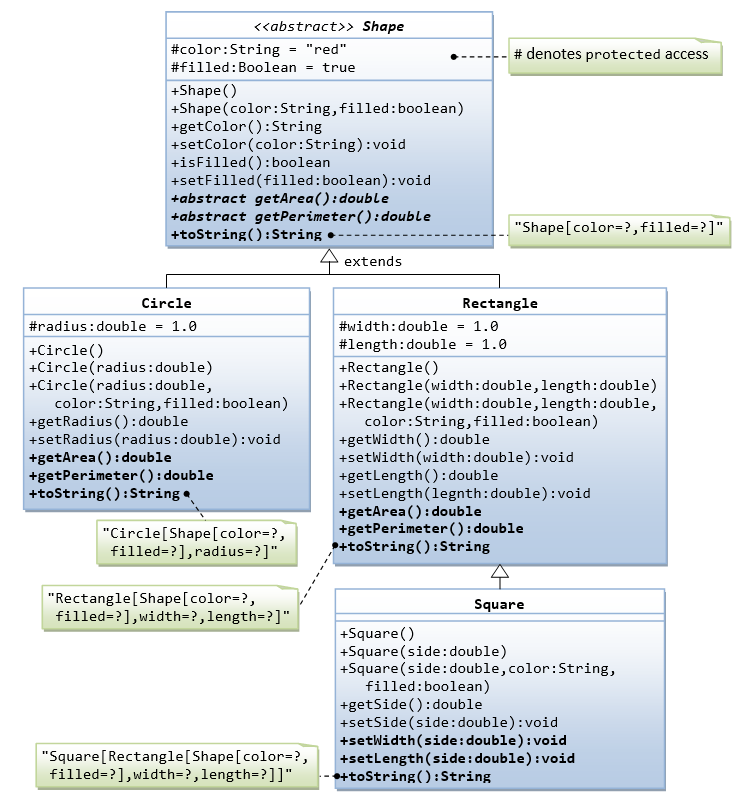In this exercise, `Shape` shall be defined as an `abstract` class, which contains:

• Two `protected` instance variables `color`(`String`) and `filled`(`boolean`). The `protected` variables can be accessed by its subclasses and classes in the same package. They are denoted with a `'#'` sign in the class diagram.
• Getter and setter for all the instance variables, and `toString()`.
• Two `abstract` methods `getArea()` and `getPerimeter()` (shown in italics in the class diagram).

The subclasses `Circle` and `Rectangle` shall override the `abstract` methods `getArea()` and `getPerimeter()` and provide the proper implementation. They also override the `toString()`.

Write a test class to test these statements involving polymorphism and explain the outputs. Some statements may trigger compilation errors. Explain the errors, if any.

```Shape s1 = new Circle(5.5, "red", false);  // Upcast Circle to Shape
System.out.println(s1);                    `// which version?`
System.out.println(s1.getArea());          // which version?
System.out.println(s1.getPerimeter());     // which version?
System.out.println(s1.getColor());
System.out.println(s1.isFilled());

Circle c1 = (Circle)s1;                   // Downcast back to Circle
System.out.println(c1);
System.out.println(c1.getArea());
System.out.println(c1.getPerimeter());
System.out.println(c1.getColor());
System.out.println(c1.isFilled());

Shape s2 = new Shape();

Shape s3 = new Rectangle(1.0, 2.0, "red", false);   // Upcast
System.out.println(s3);
System.out.println(s3.getArea());
System.out.println(s3.getPerimeter());
System.out.println(s3.getColor());
System.out.println(s3.getLength());

Rectangle r1 = (Rectangle)s3;   // downcast
System.out.println(r1);
System.out.println(r1.getArea());
System.out.println(r1.getColor());
System.out.println(r1.getLength());

Shape s4 = new Square(6.6);     // Upcast
System.out.println(s4);
System.out.println(s4.getArea());
System.out.println(s4.getColor());
System.out.println(s4.getSide());

// Take note that we downcast Shape s4 to Rectangle,
//  which is a superclass of Square, instead of Square
Rectangle r2 = (Rectangle)s4;
System.out.println(r2);
System.out.println(r2.getArea());
System.out.println(r2.getColor());
System.out.println(r2.getSide());
System.out.println(r2.getLength());

// Downcast Rectangle r2 to Square
Square sq1 = (Square)r2;
System.out.println(sq1);
System.out.println(sq1.getArea());
System.out.println(sq1.getColor());
System.out.println(sq1.getSide());
System.out.println(sq1.getLength());```

What is the usage of the `abstract` method and `abstract` class?

#### Ex: GeometricObject Interface and its Implementation Classes Circle and Rectangle

Write an interface called `GeometricObject`, which contains 2 `abstract` methods: `getArea()` and `getPerimeter()`, as shown in the class diagram. Also write an implementation class called `Circle`. Mark all the overridden methods with annotation `@Override`.#### Ex: Movable Interface and its Implementation MovablePoint Class

Write an interface called `Movaable`, which contains 4 `abstract` methods `moveUp()`, `moveDown()`, `moveLeft()` and `moveRight()`, as shown in the class diagram. Also write an implementation class called `MovablePoint`. Mark all the overridden methods with annotation `@Override`.#### Ex: Movable Interface and its Implementation Classes MovablePoint and MovableCircle

Write an interface called `Movaable`, which contains 4 `abstract` methods `moveUp()`, `moveDown()`, `moveLeft()` and `moveRight()`, as shown in the class diagram. Also write the implementation classes called `MovablePoint` and `MovableCircle`. Mark all the overridden methods with annotation `@Override`.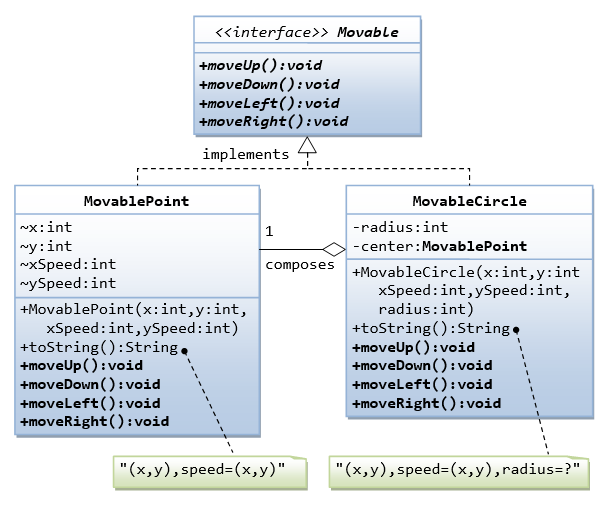#### Ex: Interfaces Resizable and GeometricObject1. Write the `interface` called `GeometricObject`, which declares two `abstract` methods: `getParameter()` and `getArea()`, as specified in the class diagram.
Hints:
```public interface GeometricObject {
public double getPerimeter();
......
}```
2. Write the implementation class `Circle`, with a protected variable `radius`, which implements the interface `GeometricObject`.
Hints:
```public class Circle implements GeometricObject {
// Private variable
......

// Constructor
......

// Implement methods defined in the interface GeometricObject
@Override
public double getPerimeter() { ...... }

......
}```
3. Write a test program called `TestCircle` to test the methods defined in `Circle`.
4. The class `ResizableCircle` is defined as a subclass of the class `Circle`, which also implements an interface called `Resizable`, as shown in class diagram. The interface `Resizable` declares an `abstract` method `resize()`, which modifies the dimension (such as `radius`) by the given percentage. Write the interface `Resizable` and the class `ResizableCircle`.
Hints:
```public interface `Resizable` {
public double resize(...);
}```
```public class `ResizableCircle` extends Circle implements Resizeable {

// Constructor
super(...);
}

// Implement methods defined in the interface Resizable
@Override
public double resize(int percent) { ...... }
}```
5. Write a test program called `TestResizableCircle` to test the methods defined in `ResizableCircle`.

#### Ex: Abstract Superclass Animal and its Implementation Subclasses

Write the codes for all the classes shown in the class diagram. Mark all the overridden methods with annotation @Override.#### Ex: Another View of Abstract Superclass Animal and its Implementation Subclasses

Examine the following codes and draw the class diagram.

```abstract public class Animal {
abstract public void greeting();
}```
```public class Cat extends Animal {
@Override
public void greeting() {
System.out.println("Meow!");
}
}```
```public class Dog extends Animal {
@Override
public void greeting() {
System.out.println("Woof!");
}

public void greeting(Dog another) {
System.out.println("Woooooooooof!");
}
}```
```public class BigDog extends Dog {
@Override
public void greeting() {
System.out.println("Woow!");
}

@Override
public void greeting(Dog another) {
System.out.println("Woooooowwwww!");
}
}```

Explain the outputs (or error) for the following test program.

```public class TestAnimal {
public static void main(String[] args) {
// Using the subclasses
Cat cat1 = new Cat();
cat1.greeting();
Dog dog1 = new Dog();
dog1.greeting();
BigDog bigDog1 = new BigDog();
bigDog1.greeting();

// Using Polymorphism
Animal animal1 = new Cat();
animal1.greeting();
Animal animal2 = new Dog();
animal2.greeting();
Animal animal3 = new BigDog();
animal3.greeting();
Animal animal4 = new Animal();

// Downcast
Dog dog2 = (Dog)animal2;
BigDog bigDog2 = (BigDog)animal3;
Dog dog3 = (Dog)animal3;
Cat cat2 = (Cat)animal2;
dog2.greeting(dog3);
dog3.greeting(dog2);
dog2.greeting(bigDog2);
bigDog2.greeting(dog2);
bigDog2.greeting(bigDog1);
}
}```

#### Ex: Interface Movable and its implementation subclasses MovablePoint and MovableCircle

Suppose that we have a set of objects with some common behaviors: they could move up, down, left or right. The exact behaviors (such as how to move and how far to move) depend on the objects themselves. One common way to model these common behaviors is to define an interface called `Movable`, with `abstract` methods `moveUp()`, `moveDown()`, `moveLeft()` and `moveRight()`. The classes that implement the `Movable` interface will provide actual implementation to these `abstract` methods.

Let's write two concrete classes - `MovablePoint` and `MovableCircle` - that implement the Movable interface.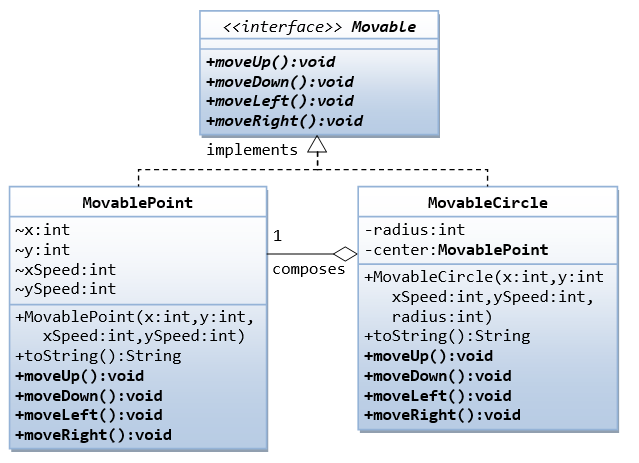The code for the interface `Movable` is straight forward.

```public interface Movable {  // saved as "Movable.java"
public void moveUp();
......
}```

For the `MovablePoint` class, declare the instance variable `x`, `y`, `xSpeed` and `ySpeed` with package access as shown with `'~'` in the class diagram (i.e., classes in the same package can access these variables directly). For the `MovableCircle` class, use a `MovablePoint` to represent its center (which contains four variable `x`, `y`, `xSpeed` and `ySpeed`). In other words, the `MovableCircle` composes a `MovablePoint`, and its `radius`.

```public class MovablePoint implements Movable { // saved as "MovablePoint.java"
// instance variables
int x, y, xSpeed, ySpeed;     // package access

// Constructor
public MovablePoint(int x, int y, int xSpeed, int ySpeed) {
this.x = x;
......
}
......

// Implement abstract methods declared in the interface Movable
@Override
public void moveUp() {
y -= ySpeed;   // y-axis pointing down for 2D graphics
}
......
}```
```public class MovableCircle implements Movable { // saved as "MovableCircle.java"
// instance variables
private MovablePoint center;   // can use center.x, center.y directly
//  because they are package accessible

// Constructor
public MovableCircle(int x, int y, int xSpeed, int ySpeed, int radius) {
// Call the MovablePoint's constructor to allocate the center instance.
center = new MovablePoint(x, y, xSpeed, ySpeed);
......
}
......

// Implement abstract methods declared in the interface Movable
@Override
public void moveUp() {
center.y -= center.ySpeed;
}
......
}```

Write a test program and try out these statements:

```Movable m1 = new MovablePoint(5, 6, 10, 15);     // upcast
System.out.println(m1);
m1.moveLeft();
System.out.println(m1);

Movable m2 = new MovableCircle(1, 2, 3, 4, 20);  // upcast
System.out.println(m2);
m2.moveRight();
System.out.println(m2);```

Write a new class called `MovableRectangle`, which composes two `MovablePoints` (representing the top-left and bottom-right corners) and implementing the `Movable` Interface. Make sure that the two points has the same speed.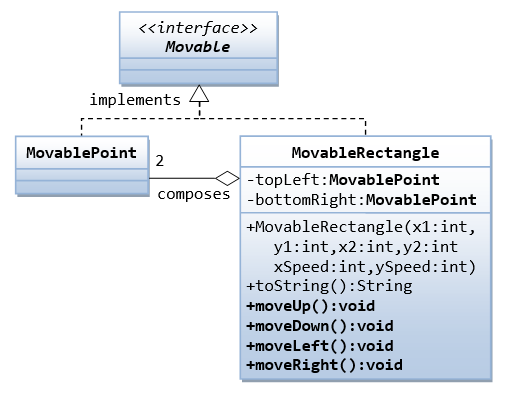What is the difference between an interface and an abstract class?

### More Exercises on OOP

#### Ex: The Discount System

You are asked to write a discount system for a beauty saloon, which provides services and sells beauty products. It offers 3 types of memberships: Premium, Gold and Silver. Premium, gold and silver members receive a discount of 20%, 15%, and 10%, respectively, for all services provided. Customers without membership receive no discount. All members receives a flat 10% discount on products purchased (this might change in future). Your system shall consist of three classes: `Customer`, `Discount` and `Visit`, as shown in the class diagram. It shall compute the total bill if a customer purchases \$x of products and \$y of services, for a visit. Also write a test program to exercise all the classes.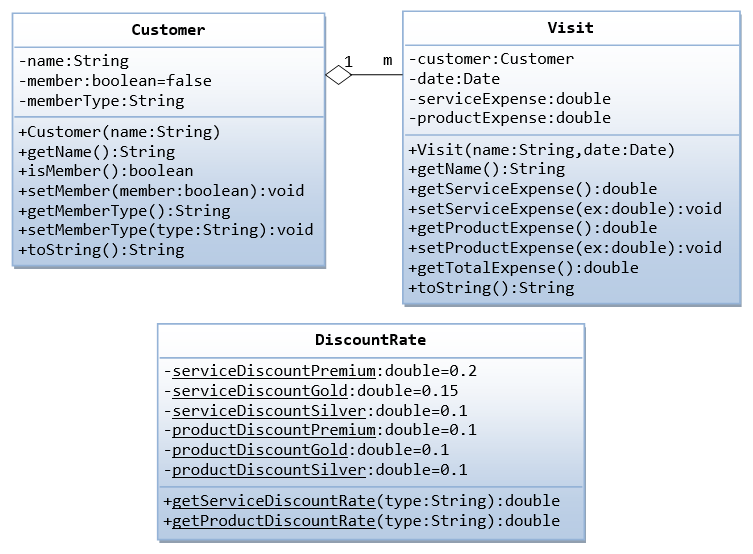The class `DiscountRate` contains only `static` variables and methods (underlined in the class diagram).

#### Ex: Polyline of Points with ArrayListA polyline is a line with segments formed by points. Let's use the `ArrayList` (dynamically allocated array) to keep the points, but upcast to `List` in the instance variable. (Take note that array is of fixed-length, and you need to set the initial length).

```public class Point {
private int x;
private int y;
public Point(int x, int y) { ...... }
public int getX() { ...... }
public int getY() { ...... }
public void setX(int x) { ...... }
public void setY(int y) { ...... }
public int[] getXY() { ...... }
public void setXY(int x, int y) { ...... }
public String toString() { ...... }
public double distance(Point another) { ...... }
}```
```import java.util.*;
public class PolyLine {
private List<Point> points;   // List of Point instances

// Constructors
public PolyLine() {  // default constructor
points = new ArrayList<Point>();  // implement with ArrayList
}
public PolyLine(List<Point> points) {
this.points = points;
}

// Append a point (x, y) to the end of this polyline
public void appendPoint(int x, int y) {
Point newPoint = new Point(x, y);
}

// Append a point instance to the end of this polyline
public void appendPoint(Point point) {
}

// Return {(x1,y1)(x2,y2)(x3,y3)....}
public String toString() {
// Use a StringBuilder to efficiently build the return String
StringBuilder sb = new StringBuilder("{");
for (Point aPoint : points) {
sb.append(aPoint.toString());
}
sb.append("}");
return sb.toString();
}

// Return the total length of this polyline
public double getLength() { ...... }
}```
```/*
* A Test Driver for the PolyLine class.
*/
import java.util.*;
public class TestPolyLine {
public static void main(String[] args) {
// Test default constructor and toString()
PolyLine l1 = new PolyLine();
System.out.println(l1);  // {}

// Test appendPoint()
l1.appendPoint(new Point(1, 2));
l1.appendPoint(3, 4);
l1.appendPoint(5, 6);
System.out.println(l1);  // {(1,2)(3,4)(5,6)}

// Test constructor 2
List<Point> points = new ArrayList<Point>();
PolyLine l2 = new PolyLine(points);
System.out.println(l2);  // {(11,12)(13,14)}
}
}```

### Exercises on Data Structures

#### Ex: MyIntStack

A stack is a first-in-last-out queue. Write a program called `MyIntStack`, which uses an array to store the contents, restricted to `int`.Write a test program.

 ```1 2 3 4 5 6 7 8 9 10 11 12 13 14 15 16 17 18 19 20 21 22 23 24 25 26 27 28 29 30``` ```public class MyIntStack { private int[] contents; private int tos; // Top of the stack // constructors public MyIntStack(int capacity) { contents = new int[capacity]; tos = -1; } public void push(int element) { contents[++tos] = element; } public int pop() { return contents[tos--]; } public int peek() { return contents[tos]; } public boolean isEmpty() { return tos < 0; } public boolean isFull() { return tos == contents.length - 1; } }```

Try:

1. Modify the `push()` method to throw an `IllegalStateException` if the stack is full.
2. Modify the `push()` to return `true` if the operation is successful, or `false` otherwise.
3. Modify the `push()` to increase the capacity by reallocating another array, if the stack is full.

Exercise (Nodes, Link Lists, Trees, Graphs):

[TODO]

• Study the existing open source codes, including JDK.
• Specialized algorithms, such as shortest path.

Exercise (Maps):

[TODO]

• Representation of map data.
• Specialized algorithms, such as shortest path.

Exercise (Matrix Operations for 3D Graphics):

[TODO]

• Study the existing open source codes, including JDK's 2D Graphics and JOGL's 3D Graphics.
• Efficient and specialized codes for 3D Graphics (4D matrices). Handle various primitive types such as `int`, `float` and `double` efficiently.